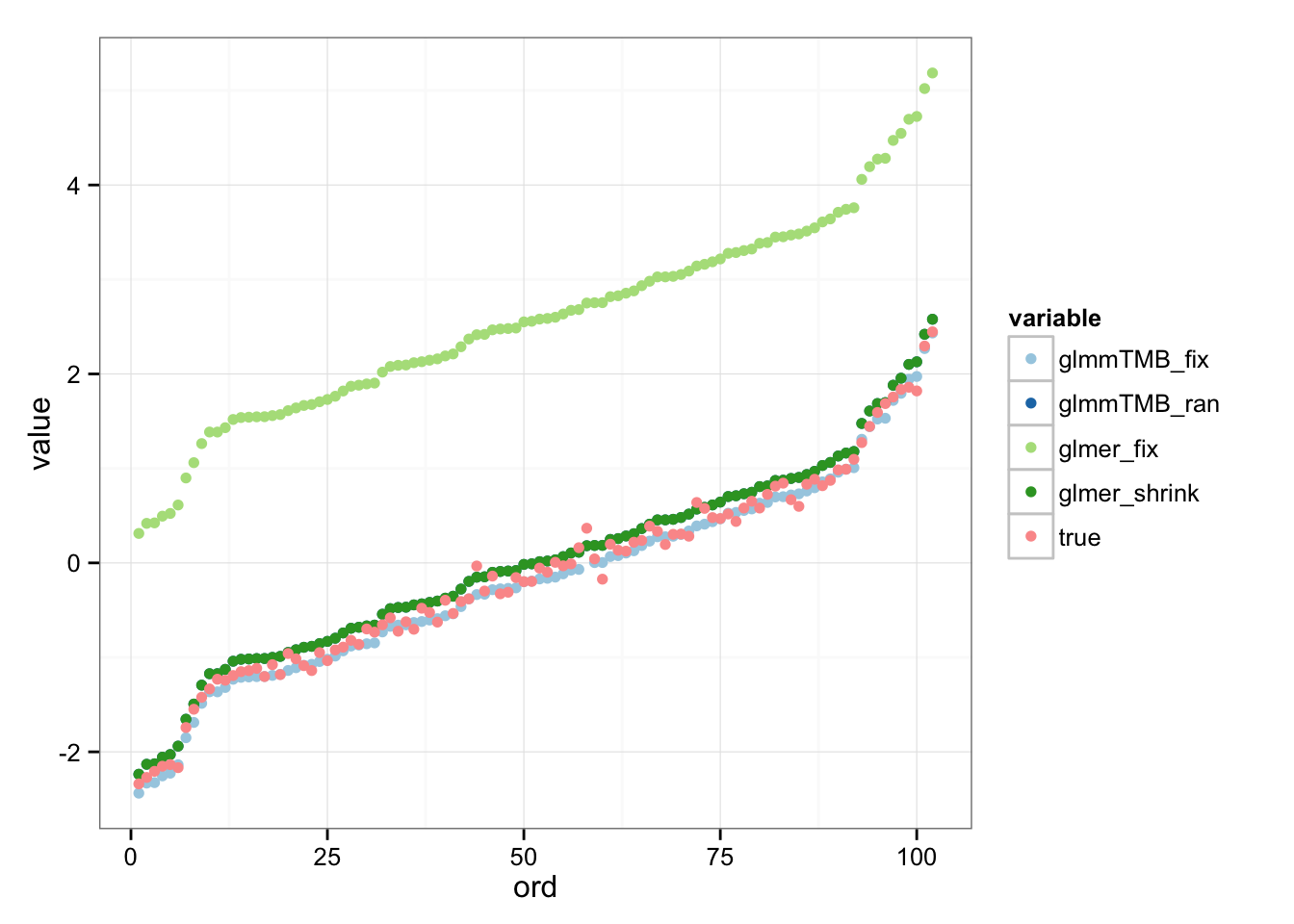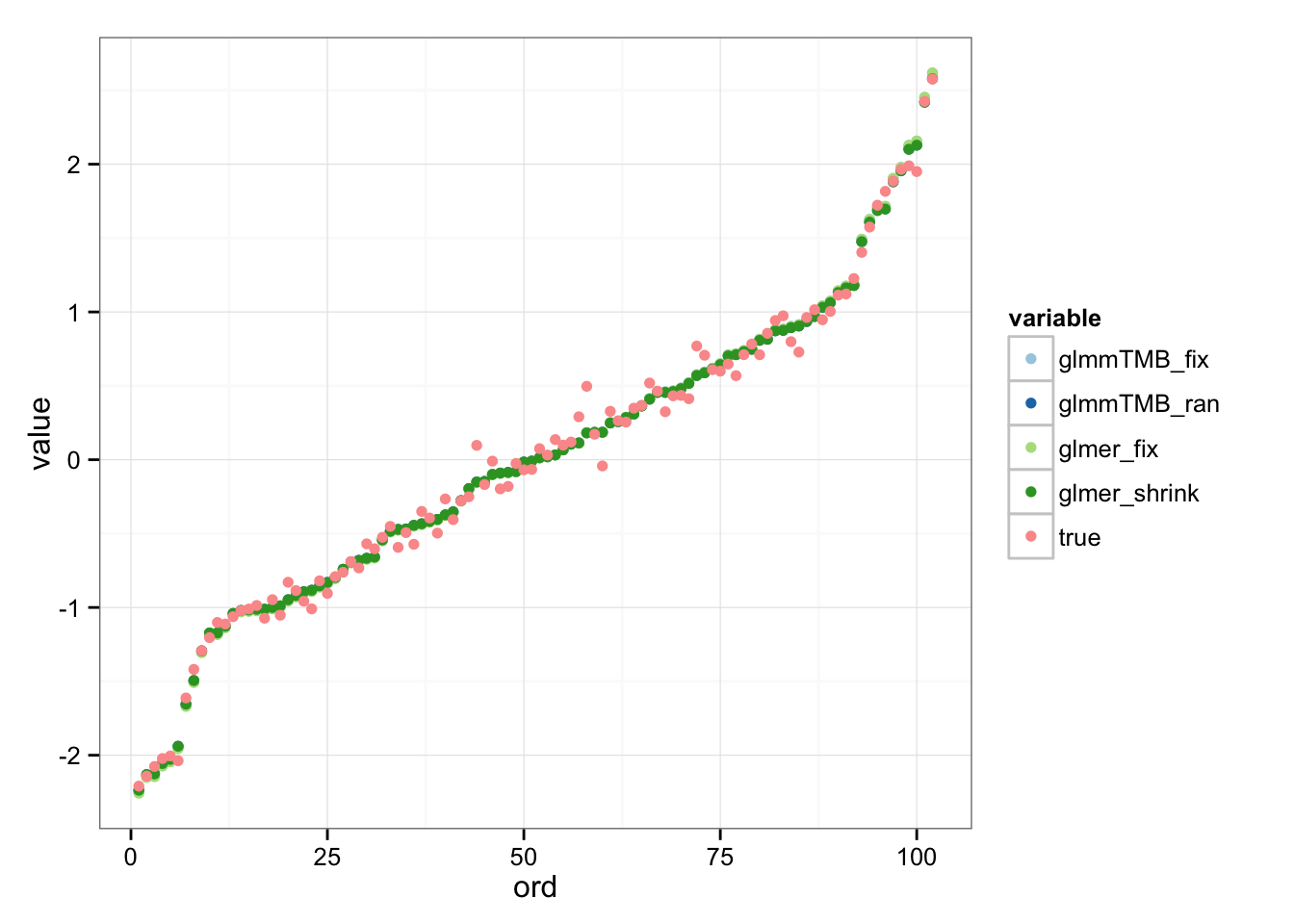A reasonably frequent question (it’s come up at least a couple of times in the last few years) is how to fit models with categorical predictors that have many levels. This case is hard for two reasons:

• `lme4`’s approach doesn’t scale terribly well for GLMMs with large numbers of fixed-effect parameters (for GLMMs, unless `nAGQ==0`, the fixed effect parameters and the “theta” (log-Cholesky parameterization of the random effects variance-covariance matrices) parameters are combined in a single nonlinear optimization step).
• current versions of `lme4` don’t use sparse matrices for the fixed-effect model matrix `X`. If there are categorical predictors with large numbers of categories, the number of columns in `X`, and hence its size, explodes.
``````library("lme4")
library("ggplot2"); theme_set(theme_bw())
library("reshape2")
library("plyr")``````

Set up data:

``````n <- 1.5e5 ## start a bit small ...
ncat <- 102
ngrp <- 25
set.seed(101)
dd <- data.frame(x=rnorm(n),
y=sample(letters[1:3],replace=TRUE,size=n),
z=factor(sample(1:ncat,replace=TRUE,size=n)),
ID=factor(sample(1:ngrp,replace=TRUE,size=n)))
beta0 <- with(dd,rnorm(1+1+length(unique(y))-1+length(unique(z))-1))
form <- outcome ~ x + y + z + (1|ID)
dd\$outcome <- suppressMessages(simulate(form[-2],
newdata=dd,
newparams=list(theta=2,beta=beta0),
weights=rep(1,n),
family=binomial)[])``````

Now fit the model. This more or less follows the framework set out in `?modular`, but it requires a few hacks along the way (a few more than I would have hoped …)

``````t.start <- Sys.time()
m1 <- glFormula(outcome ~ x + y + (1|z) + (1 |   ID), data = dd,
family = "binomial")
devfun0 <- do.call(mkGlmerDevfun,m1)
## write a wrapper:
## lme4 organizes RE terms in *decreasing* order of number
## of levels of grouping variable
devfun <- function(theta,zsd=1000) {
devfun0(c(zsd,theta))
}
## make sure environments are set appropriately
environment(devfun) <- environment(devfun0)
## optimize stage 1
## (lower=0 might need to be adjusted for a more complex model)
opt1 <- nloptwrap(devfun,par=1,zsd=1000,lower=0,upper=Inf)
devfun2 <- updateGlmerDevfun(devfun0,m1\$reTrms)
## set up a new wrapper, with appropriate environment
devfun3 <- function(p,zsd=1000) {
devfun2(c(zsd,p))
}
environment(devfun3) <- environment(devfun2)
## fit stage 2:
nbeta <- ncol(m1\$X)
ntheta <- length(opt1\$par)
## testing: devfun3(c(opt1\$par,rep(0,nbeta)))
opt2 <- nloptwrap(devfun3,par=c(opt1\$par,rep(0,nbeta)),
lower=c(rep(0,ntheta),rep(-Inf,nbeta)),
upper=rep(Inf,ntheta+nbeta),
zsd=1000)
## package the results
res <- mkMerMod(environment(devfun3), opt2, m1\$reTrms, fr=m1\$fr)
res@beta <- opt2\$par[-1] ## ugh; mkMerMod is confused about # beta params
Sys.time()-t.start``````
``## Time difference of 50.41371 secs``

50 seconds (on a reasonably new MacBook Pro) is not as fast as I’d like, but doesn’t seem totally unreasonable …

Compare to

``````system.time(shrink.model <- glmer(outcome ~ x + y + (1|z) + (1 |   ID),
data = dd,
family = "binomial"))``````
``````##    user  system elapsed
## 105.048  10.633 116.046``````
``res``
``````## Generalized linear mixed model fit by maximum likelihood (Laplace
##   Approximation) [glmerMod]
##  Family: binomial  ( logit )
##       AIC       BIC    logLik  deviance  df.resid
##  84855.88  84915.39 -42421.94  84843.88    149994
## Random effects:
##  Groups Name        Std.Dev.
##  z      (Intercept) 1000.000
##  ID     (Intercept)    2.763
## Number of obs: 150000, groups:  z, 102; ID, 25
## Fixed Effects:
## (Intercept)            x           yb           yc
##     -0.1659      -2.0219      -0.3601       0.3485``````
``str(rr <- ranef(res))``
``````## List of 2
##  \$ z :'data.frame':  102 obs. of  1 variable:
##   ..\$ (Intercept): num [1:102] 2.75 1.71 3.47 3.45 1.55 ...
##  \$ ID:'data.frame':  25 obs. of  1 variable:
##   ..\$ (Intercept): num [1:25] 1 6.62 -1.51 3.29 -3 ...
##  - attr(*, "class")= chr "ranef.mer"``````
``````## z parameters ... (0-mean parameterization)
``##  2.750790 1.705983 3.470289 3.451865 1.546169 3.546540``

## glmmTMB

The glmmTMB package should not have as many scaling restrictions (although in its current form it does still construct a dense `X` matrix …)

``library("glmmTMB")``
``````system.time(m2 <- glmmTMB(outcome ~ x + y + z + (1 |   ID), data = dd,
family = "binomial"))``````
``````##    user  system elapsed
## 140.693   6.980 148.940``````
``````system.time(m2shrink <- glmmTMB(outcome ~ x + y + (1 | z) + (1 |   ID),
data = dd,
family = "binomial"))``````
``````##    user  system elapsed
##  51.188   2.591  54.161``````

Comparison: